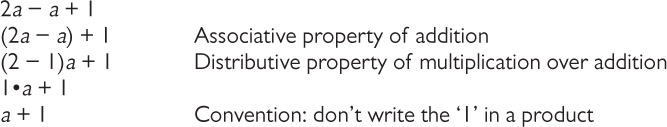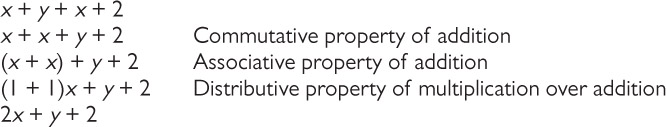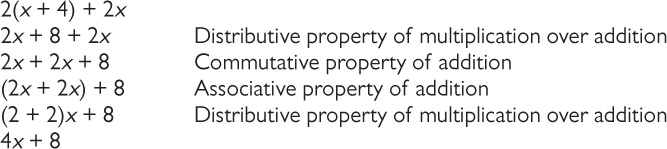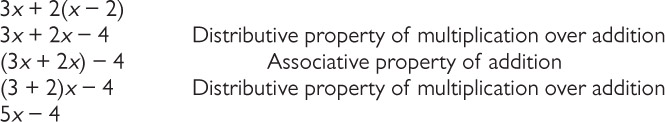Subject:
Algebra
Material Type:
Lesson Plan
Level:
Middle School
6
Provider:
Pearson
Tags:
Language:
English
Media Formats:
Interactive, Text/HTML

# Using Variables to Represent Measurements## Overview

Students write an expression for the length of a train, using variables to represent the lengths of the different types of cars.

# Key Concepts

• A numerical expression consists of a number or numbers connected by the arithmetic operations of addition, subtraction, multiplication, division, and exponentiation.
• An algebraic expression uses letters to represent numbers.
• An algebraic expression can be written to represent a problem situation. Sometimes more than one algebraic expression may represent the same problem situation. These algebraic expressions have the same value and are equivalent.
• The properties of operations can be used to make long algebraic expressions shorter:
• The commutative property of addition states that changing the order of the addends does not change the end result:
a + b = b + a.
• The associative property of addition states that changing the grouping of the addends does not change the end result:
(a + b) + c = a + (b + c).
• The distributive property of multiplication over addition states that multiplying a sum by a number gives the same result as multiplying each addend by the number and then adding the products together:
a(b + c) = ab + ac.

# Goals and Learning Objectives

• Write algebraic expressions that describe lengths of freight trains.
• Use properties of operations to shorten those expressions.

# Lesson Guide

Have students watch the train video. Students may need to view the video more than once.

• What kinds of railroad cars did you notice in this video? [Answers: locomotive, flatcar, boxcar]
• Suppose the length of the locomotive is a, the length of the flatcar is b, and the length of the boxcar is c. How can you express the length of the train?

Have students discuss how they would represent the length of the train. Elicit the fact that one way to represent the length of the train is to write the letters that represent the length of each car in the order of the cars that make up the train.

a + b + c + c + b + c

Ask students: How could you use the properties of operations to shorten the expression a + b + c + c + b + c?

Elicit the following:

a + b + c + c + b + c =

a + b + b + c + c + c =

a + 2b + 3c

Point out that students have just expressed the length of the train using an expression. Call attention to the fact that, following the convention, we have written a, not 1a.

Briefly discuss the term expression. Make sure students understand that an expression consists of numbers connected by the arithmetic operations of addition, subtraction, multiplication, division, and exponentiation.

ELL: When showing the video, be sure that ELLs can follow the explanations. “Chunk” the video by pausing at key times to allow ELLs time to process the information. Show the video again if needed. Ask questions to check for understanding before moving on to the discussion. If you notice ELLs do not understand the important parts of the video, show it one more time.

When introducing new words or using words that students recently learned (whose meaning they may have forgotten), write them down, if possible. Ask some of the students to repeat after you. Be sure ELLs feel comfortable with the pronunciation. Make sure ELLs understand the task at hand and the vocabulary contained in the questions.

# Train Cars

Think and Discuss

• Watch the video of a train. Pay attention to the different types of cars that make up the train.
• Suppose the train has two flatcars. If the length of a flatcar is some number b, then the length of two flatcars can be represented as b + b, or 2b.

VIDEO: Train Cars

# Lesson Guide

Discuss the Math Mission. Students will write an expression for the length of a train, using variables to represent the lengths of the different types of cars.

## Opening

Express the lengths of various trains using numbers, letters, and operations.

# Lesson Guide

Have students work individually to make several trains and write expressions for their trains. Let students work individually or with a partner on their presentations for Task 4.

# Mathematical Practices

Mathematical Practice 2: Reason abstractly and quantitatively.

As students create expressions for their trains, they are representing each type of car abstractly using a different variable. Look for students who use multiple variables in their expressions and who can explain what their expressions represent.

Mathematical Practice 8: Look for and express regularity in repeated reasoning.

Look for students who are able to shorten their expressions using different properties and then conclude that they can combine like terms.

# Interventions

Student has difficulty getting started.

• What cars can you use to make your train? How many cars do you need to use?
• How will you represent a car’s length?
• What operation will you use in the expression for your train’s length? Explain how you know this.
• Is there a way to make your expression shorter? Explain your thinking.

Student has a solution.

• Describe how you wrote the expression for the length of your train.
• What do the variables represent?
• Explain how you knew what operation to use in your expression.
• Can you make your expression shorter? Explain why or why not.
• What properties did you use to shorten the expression for your train’s length? Explain what you did.
• Do you think it is easier to work with long expressions or short expressions? Explain your thinking.

• Trains will vary. Possible answer: My train has 1 locomotive, 1 flatcar, 3 boxcars, 2 tank cars, and 1 caboose.
• Expressions will vary. Possible answer: a + b + c + c + c + d + d + e, or a + b + 3c + 2d + e
• Trains and expressions will vary. Check students’ work.
• Expressions and trains will vary.

# Make a Train

• Use the interactive to make a train that has at least 8 cars.
• Write an expression to represent the train. Write the expression again in a different form.
• Make several other trains, and write expressions for them.
• Give one of your expressions to your partner, and challenge your partner to create the corresponding train.

INTERACTIVE: Creating Trains

## Hint:

For each car that you put in your train, add a letter to your expression that represents the length of the car.

# Preparing for Ways of Thinking

As students work, look for these approaches:

• Expresses the length of the train by listing each car length in the order it appears on the train:
a + c + c + b + c + b + b + e
• Uses the distributive property to list groups of similar lengths in the order they appear on the train:
a + 2c + b + c + 2b + e
• Uses the commutative, associative, and distributive properties to express the length of each train:
a + 3b + 3c + e

# Challenge Problem

• Answers will vary. Check students’ work.

# Prepare a Presentation

Prepare a presentation that shows how you wrote expressions to represent your trains and how you created a train given your partner’s expression. Explain your thinking.

# Challenge Problem

• Make a different train for the expression you wrote to represent one of the trains you made. How is it similar to your original train? How is it different?

# Lesson Guide

As students share their work, ask them to be explicit about how the properties are used to write shorter expressions. For example, the commutative and associative properties of addition are used to express the length of the train a + c + c + b + c + b + b + e as a + 3b + 3c + e.

• What cars did you use for your train? What expression did you write?
• What do the letters in your expression represent?
• What mathematical symbols did you use in your expression? Why?
• What does this part of your expression represent?
• Explain what the associative, commutative, and distributive properties mean.
• How did you make the expression for your train shorter? What properties did you use?
• How can you shorten a + a? How do you know?
• What does a number in front of a letter mean?
• Does someone have another example of how they shortened the expression for their train?

Display student work that shows expressions written as short as possible and work that shows expressions that can still be shortened. When a student shows an expression that can be shortened, have the class work together to shorten it.

• Can any of these expressions be shortened? Explain your thinking.

As you contrast and compare the different expressions for each train, ask students which expressions might be easier to work with and why.

• Look at [Name]’s expression and [Name]’s expression.
• What do you notice about these expressions?
• Which expression might be easier to work with? Explain your thinking.

ELL: ELLs may struggle to follow the discussion and concurrently take notes during the Ways of Thinking portion of the lesson. Create notes or questions based on the presentations for some students to use as reference and support during the Ways of Thinking portion of the lesson.

# Ways of Thinking: Make Connections

Takes notes about your classmates' approaches to making trains, writing expressions, and making trains to match expressions.

## Hint:

• What cars did you use in your train?
• Can you explain how you rewrote your expression?
• Can you make your expression shorter?
• What strategy did you use for making a train based on the expression your partner gave you?
• To make a train based on an expression, which do you think is easier to work with, the original expression or the rewritten expression? Why?

# Lesson Guide

Ask questions such as the following as students are working:

• How did you rewrite this expression? Explain the steps you used.
• What properties did you use to rewrite this expression?
• Why can you combine these terms?
• Can you rewrite this expression any shorter?

SWD: If students seem unsure of the task, model the steps for solving the problem with them before asking them to do the problems themselves.

1.2.3.4.# Rewriting Expressions

Rewrite these expressions to combine like terms. State what property of operations you used in rewriting each step.

1. 2aa + 1
2. x + y + x + 2
3. 2(x + 4) + 2x
4. 3x + 2(x − 2)

## Hint:

• In the expression 3+a, how many of a do you have? Can you put a number in front ofa ?
• How do you distribute 2 over x+4? In other words, how can you rewrite 2(x+4)?
• You cannot add 2x and 8 to get 10x. Why?

The properties of operations are:

• Associative property of addition: (a+b)+c=a+(b+c)
• Commutative property of addition: a+b=b+a
• Additive identity property of 0: a+0=0+a=a
• Associative property of multiplication: (a×b)×c=a×(b×c)
• Commutative property of multiplication: a×b=b×a
• Multiplicative identity property of 1: a×1=1×a=a
• Multiplicative inverse property: 1a×a=1.
• Distributive property of multiplication over addition: a×(b+c)=a×b+a×c

# Lesson Guide

• Have pairs quietly discuss the information in the Summary.
• As students work together in pairs, identify any misunderstandings students may still have. Make a note to address these issues in the class discussion.
• Discuss the Summary as a class. Include the following points:
• You can express the length of a freight train as the sum of the lengths of each car, and you can use variables to represent the lengths of the cars.
• The commutative property of addition states that changing the order of the addends does not change the end result: a + b = b + a.
• The associative property of addition states that changing the grouping of the addends does not change the end result: (a + b) + c = a + (b + c).
• The distributive property of multiplication over addition states that multiplying a sum by a number gives the same result as multiplying each addend by the number and then adding the products together: a(b + c) = ab + ac.

# Summary of the Math: Understand Expressions

• In an expression, the letter a represents any number. The letter a is called a variable.
• When you add two numbers, the sum is another number. When you add any number a to 3, the sum is a + 3. Since a represents a number, a + 3 also represents a number. If you add a + a you get 1a + 1a or (1 + 1)a or 2a. You have just used the distributive property.
• When you multiply a by 3, you write the product as 3a instead of 3 × a, 3 · a, × 3, a · 3, or a3. Since a represents a number, 3a also represents a number.
• 3a and a + 3 are called expressions. To be exact, they are variable expressions or algebraic expressions. An algebraic expression uses mathematical symbols, numbers, and/or letters to express a value.

## Hint:

Can you:

• Explain how to represent the length of the train using numbers, letters, and operations?
• Write an expression that involves addition?
• Use the distributive property to add b+b?